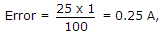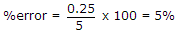# Electronics and Communication Engineering - Measurements and Instrumentation - Discussion

2.

An ammeter of 0-25 A range has a guaranteed accuracy of 1% of full scale reading. The current measured is 5 A. The limiting error is

 [A]. 2% [B]. 2.5% [C]. 4% [D]. 5%

Explanation:.

 Imran said: (Apr 13, 2014) 0.25/5 = 0.05x100 = 5%.

 Anil said: (May 4, 2014) Please correct it. % error = 0.25*100/5volt = 5%.

 Sanju said: (Jul 6, 2014) Scale given 1% so 25x1/100 = 0.25A. %error = 0.25/58x100 = 5%.

 Shinykiran said: (Dec 10, 2015) How the value 58 came?

 Sharada said: (Aug 21, 2016) What is the formula used here? Can anyone say?

 Bindu said: (Apr 27, 2017) I am not understanding. Can anyone explain properly?

 Rajeev said: (May 25, 2017) Here, (% error at desired scale * desired scale ) = (%error at full scale value * full scale value).

 Mahesh Thapa Magar said: (Jul 21, 2017) %Error(DS) * DS = %Error(FS) * FS. DS:Desired scale. FS:Full SCale.

 Mangai said: (Jul 21, 2017) Limiting error = accuracy * full scale reading = (1%) * 25 = (1/100) *25 = 0.25 A error in percentage. % error=(maximum error/scale reading) * 100 = (0.25/ 5) *100 = 5%.

 Narender said: (Feb 26, 2018) 1/25*100=4, 4/100*5=20/100, = 5%.

 Hasnain Khizar said: (Mar 12, 2018) @All. It is actually the conversion from absolute and percentage error. The key is to understand them properly and especially their conversions and where to use which one.

 Rayal Jp said: (Dec 19, 2018) (M.v-T.v/T.v) * 100.